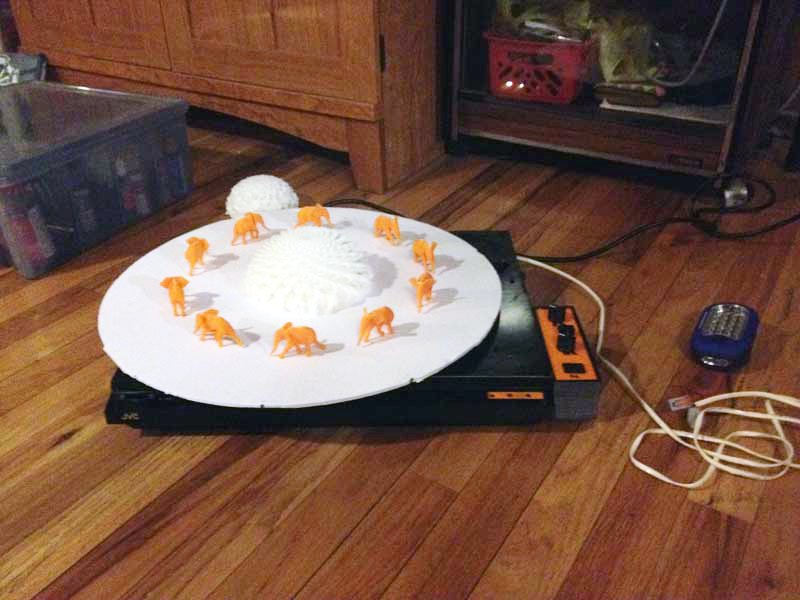# 6. 3D Turn-o-trope – Arduino Code

```0. Introduction

1. Basic Operation

2. The Conversion Overview

3. The Conversion Details

4. Main Components

5. Schematics```

# 5. 3D Turn-o-trope – Schematics

```0. Introduction

1. Basic Operation

2. The Conversion Overview

3. The Conversion Details

4. Main Components

6. Arduino Code```

# 4. 3D Turn-o-trope – Main Components

```0. Introduction

1. Basic Operation

2. The Conversion Overview

3. The Conversion Details

5. Schematics

6. Arduino Code```

# 3. 3D Turn-o-trope – The Conversion Details

```0. Introduction

1. Basic Operation

2. The Conversion Overview

4. Main Components

5. Schematics

6. Arduino Code```

# 2. 3D Turn-o-trope – The Conversion Overview

```0. Introduction

1. Basic Operation

3. The Conversion Details

4. Main Components

5. Schematics

6. Arduino Code```

# 1. 3D Turn-o-trope – Basic Operation

```0. Introduction

2. The Conversion Overview

3. The Conversion Details

4. Main Components

5. Schematics

6. Arduino Code```

# 0. 3D Turn-o-trope – Introduction

A project to convert an old record player into a controllable turntable for physical 3D animations.```1. Basic Operation

2. The Conversion Overview

3. The Conversion Details

4. Main Components

5. Schematics

6. Arduino Code
```